Theoretical and Computational Details of the Semivariogram

Letbe a spatial random field (SRF) withmeasured values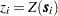at respective locations,. You use the VARIOGRAM procedure because you want to gain insight into the spatial continuity and structure of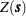. A good measure of the spatial continuity ofis defined by means of the variance of the difference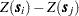, whereand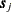are locations in. Specifically, if you considerandto be spatial increments such that, then the variance function based on the incrementsis independent of the actual locations,. Most commonly, the continuity measure used in practice is one half of this variance, better known as the semivariance function,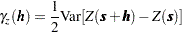or, equivalently,The plot of semivariance as a function ofis the semivariogram. You might also commonly see the term semivariogram used instead of the term semivariance.

Assume that the SRFis free of nonrandom (or systematic) surface trends. Then, the expected value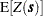ofis a constant for all, and the semivariance expression is simplified to the following: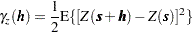Given the preceding assumption, you can compute an estimateof the semivariance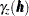from a finite set of points in a practical way by using the formulawhere the setscontain all the neighboring pairs at distance,and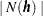is the number of such pairs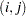.

The expression foris called the empirical semivariance (Matheron; 1963). This is the quantity that PROC VARIOGRAM computes, and its corresponding plot is the empirical semivariogram.

The empirical semivarianceis also referred to as classical. This name is used so that it can be distinguished from the robust semivariance estimate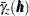and the corresponding robust semivariogram. The robust semivariance was introduced by Cressie and Hawkins (1980) to weaken the effect that outliers in the observations might have on the semivariance. It is described by Cressie (1993, p. 75) as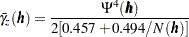In the preceding expression the parameter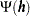is defined asAccording to Cressie (1985), the estimatehas approximate variance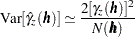This approximation is possible by assumingto be a Gaussian SRF, and by further assuming the squared differences in empirical semivariances to be uncorrelated for different distances. Typically, semivariance estimates are correlated because of the underlying spatial correlation among the observations, and also because the same observation pairs might be used for the estimation of more than one semivariogram point, as described in the following subsections. Despite these restrictive assumptions, the approximate variance provides an idea about the semivariance estimate variance and enables fitting of a theoretical model to the empirical semivariance; see the section Theoretical Semivariogram Model Fitting for more details about the fitting process.

Note: If your data include a surface trend, then the empirical semivarianceis not an estimate of the theoretical semivariance function. Instead, rather than the spatial increments variance, it represents a different quantity known as pseudo-semivariance, and its corresponding plot is a pseudo-semivariogram. In principle, pseudo-semivariograms do not provide measures of the spatial continuity. They can thus lead to misinterpretations of thespatial structure, and are consequently unsuitable for the purpose of spatial prediction. For further information, see the detailed discussion in the section Empirical Semivariograms and Surface Trends. Under certain conditions you might be able to gain some insight about the spatial continuity with a pseudo-semivariogram. This case is presented in Analysis without Surface Trend Removal.